# USING IMPEDANCE AND ADMITTANCEClick or Tap the Example circuits below to invoke TINACloud and select the Interactive DC mode to Analyze them Online.
Get a low cost access to TINACloud to edit the examples or create your own circuitsPASSIVE COMPONENTS IN AC CIRCUITS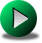VOLTAGE AND CURRENT DIVISION

As we saw in the previous chapter, impedance and admittance can be manipulated using the same rules as are used for DC circuits. In this chapter we will demonstrate these rules by calculating total or equivalent impedance for series, parallel and series-parallel AC circuits.

Example 1

Find the equivalent impedance of the following circuit:

R = 12 ohm, L = 10 mH, f = 159 Hz

Click/tap the circuit above to analyze on-line or click this link to Save under WindowsThe elements are in series, so we realise that their complex impedances should be added:

Zeq = ZR + ZL = R + j w L = 12 + j*2*p*159*0.01 = (12 + j 9.99) ohm = 15.6 ej39.8Â° ohm.

Yeq = 1/Zeq = 0.064 e- j 39.8Â° S = 0.0492 â€“ j 0.0409 S

We can illustrate this result using impedance meters and the Phasor Diagram in
TINA v6. Since TINAâ€™s impedance meter is an active device and we are going to use two of them, we must arrange the circuit so that the meters donâ€™t influence each other.
We have created another circuit just for the measurement of the part impedances. In this circuit, the two meters do not â€śseeâ€ť each otherâ€™s impedance.

The Analysis/AC Analysis/Phasor diagram command will draw the three phasors on one diagram. We used the Auto Label command to add the values and the Line command of the Diagram Editor to add the dashed auxiliary lines for the parallelogram rule.

The circuit for measuring the impedances of the parts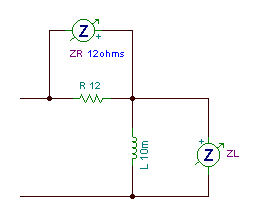Click/tap the circuit above to analyze on-line or click this link to Save under Windows

Phasor diagram showing the construction of Zeq with the parallelogram ruleAs the diagram shows, the total impedance, Zeq, can be considered as a complex resultant vector derived using the parallelogram rule from the complex impedances ZR and ZL .

Example 2

Find the equivalent impedance and admittance of this parallel circuit:

R =20 ohm, C = 5 mF, f = 20 kHz

The admittance:The impedance using the Ztot= Z1 Z2 / (Z1 + Z2 ) formula for parallel impedances: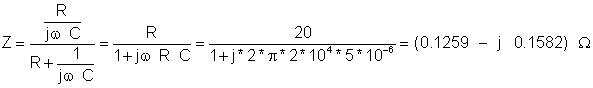Check your calculations using TINAâ€™s Analysis menu Calculate nodal voltages. When you click on the Impedance meter, TINA presents both the impedance and admittance and gives the results in algebraic and exponential forms.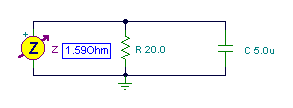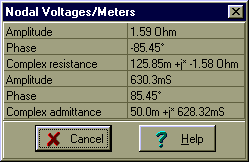Another way TINA can solve this problem is with its Interpreter:

 om:=2*pi*20000; Z:=Replus(R,(1/j/om/C)) Z=[125.8545m-1.5815*j] Y:=1/R+j*om*C; Y=[50m+628.3185m*j]

Example 3

Find the equivalent impedance of this parallel circuit. It uses the same elements as in Example 1:
R = 12 ohm and L = 10 mH, at f = 159 Hz frequency.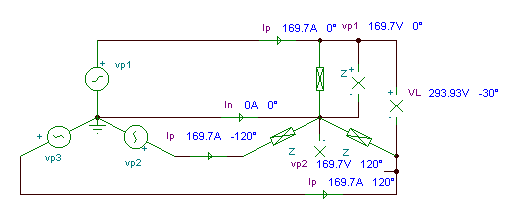For parallel circuits, itâ€™s often easier to calculate the admittance first:

Yeq = YR + YL = 1/R + 1/ (j*2*p*f*L) = 1/12 â€“ j /10 = 0.0833 â€“ j 0.1 = 0.13 e-j 50Â° S

Zeq = 1 / Yeq = 7.68 e j 50Â° ohm.

Click/tap the circuit above to analyze on-line or click this link to Save under Windows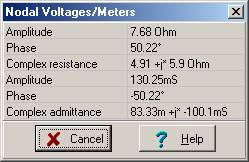Another way TINA can solve this problem is with its Interpreter:

 f:=159; om:=2*pi*f; Zeq:=replus(R,j*om*L); Zeq=[4.9124+5.9006*j]

Example 4

Find the impedance of a series circuit with R = 10 ohm, C = 4 mF, and L = 0.3 mH, at an angular frequency w = 50 krad/s (f = w / 2p = 7.957 kHz ).

Z = R + j w L - j / wC = 10 + j 5*104 * 3*10-4 â€“ j / (5*104 *4 * 10-6 ) = 10 + j 15 â€“ j 5

Z = (10 + j 10) ohm = 14.14 ej 45Â° ohms.

The circuit for measuring the impedances of the parts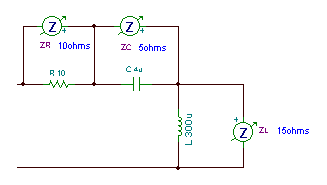Click/tap the circuit above to analyze on-line or click this link to Save under Windows

The phasor diagram as generated by TINA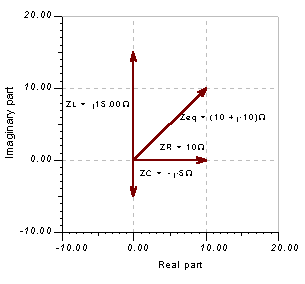Starting with the phasor diagram above, letâ€™s use the triangle or geometric construction rule to find the equivalent impedance. We start by moving the tail of ZR to the tip of ZL. Then we move the tail of ZC to the tip of ZR. Now the resultant Zeq will exactly close the polygon starting from the tail of the first ZR phasor and ending at the tip of ZC.

The phasor diagram showing the geometric construction of Zeq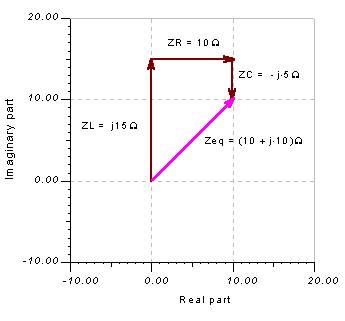{Solution by TINA's Interpreter} om:=50k; ZR:=R; ZL:=om*L; ZC:=1/om/C; Z:=ZR+j*ZL-j*ZC; Z=[10+10*j] abs(Z)=[14.1421] radtodeg(arc(Z))= {other way} Zeq:=R+j*om*L+1/j/om/C; Zeq=[10+10*j] Abs(Zeq)=[14.1421] fi:=arc(Z)*180/pi; fi=

Check your calculations using TINAâ€™s Analysis menu Calculate nodal voltages. When you click on the Impedance meter, TINA presents both the impedance and admittance, and gives the results in algebraic and exponential forms.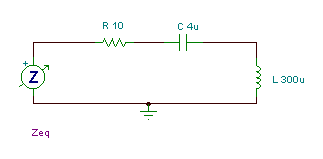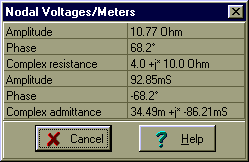Since the circuitâ€™s impedance has a positive phase like an inductor, we can call it an inductive circuit--at least at this frequency!

Example 5

Find a simpler series network that could replace the series circuit of example 4 (at the given frequency).

We noted in Example 4 that the network is inductive, so we can replace it by a 4 ohm resistor and a 10 ohm inductive reactance in series:

XL = 10 = w*L = 50*103 L

Â® L = 0.2 mH

Donâ€™t forget that, since inductive reactance depends upon frequency, this equivalence is valid only for one frequency.

Example 6

Find the impedance of three components connected in parallel: R = 4 ohm, C = 4 mF, and L = 0.3 mH, at an angular frequency w = 50 krad/s (f = w / 2p = 7.947 kHz).

Noting that this is a parallel circuit, we solve first for the admittance:

1/Z = 1/R +1/ j w L + jwC = 0.25 â€“ j/15 +j0.2 = 0.25 +j 0.1333

Z = 1/(0.25 + j 0.133) = (0.25 â€“ j 0.133)/0.0802 = 3.11 â€“ j 1.65 =3.5238 e-j 28.1Â° ohms.

 {Solution by TINA's Interpreter} om:=50k; ZR:=R; ZL:=om*L; ZC:=1/om/C; Z:=1/(1/R+1/j/ZL-1/j/ZC); Z=[3.1142-1.6609*j] abs(Z)=[3.5294] fi:=radtodeg(arc(Z)); fi=[-28.0725]

The Interpreter calculates phase in radians. If you want phase in degrees, you can convert from radians to degrees by multiplying by 180 and dividing by p. In this last example, you see a simpler wayâ€”use the Interpreterâ€™s built in function, radtodeg. There is an inverse function as well, degtorad. Note that this networkâ€™s impedance has a negative phase like a capacitor, so we say thatâ€”at this frequencyâ€”it is a capacitive circuit.

In Example 4 we placed three passive components in series, while in this example we placed the same three elements in parallel. Comparing the equivalent impedances calculated at the same frequency, reveals that they are totally different, even their inductive or capacitive character.

Example 7

Find a simple series network that could replace the parallel circuit of example 6 (at the given frequency).

This network is capacitive because of the negative phase, so we try to replace it with a series connection of a resistor and a capacitor:

Zeq = (3.11 â€“ j 1.66) ohm = Re â€“j / wCe

Click/tap the circuit above to analyze on-line or click this link to Save under Windows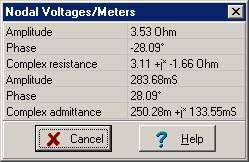Re = 3.11 ohm w*C = 1/1.66 = 0.6024

hence

Re = 3.11 ohm
C = 12.048
mF

You could, of course, replace the parallel circuit with a simpler parallel circuit in both examples

Example 8

Find the equivalent impedance of the following more complicated circuit at frequency f=50 Hz: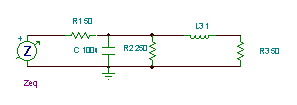Click/tap the circuit above to analyze on-line or click this link to Save under Windows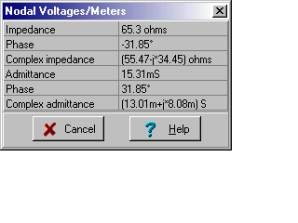{Solution by TINA's interpreter} om:=2*pi*50; Z1:=R3+j*om*L3; Z2:=replus(R2,1/j/om/C); Zeq:=R1+Replus(Z1,Z2); Zeq=[55.469-34.4532*j] abs(Zeq)=[65.2981] radtodeg(arc(Zeq))=[-31.8455]

We need a strategy before we begin. First weâ€™ll reduce C and R2 to an equivalent impedance, ZRC. Then, seeing that ZRC is in parallel with the series-connected L3 and R3, weâ€™ll compute the equivalent impedance of their parallel connection, Z2. Finally, we calculate Zeq as the sum of Z1 and Z2.

Hereâ€™s the calculation of ZRC: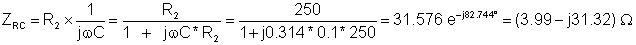Hereâ€™s the calculation of Z2: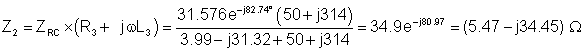And finally:

Zeq = Z1 + Z2 = (55.47 â€“ j 34.45) ohm = 65.3 e-j31.8Â° ohm

according to TINAâ€™s result.PASSIVE COMPONENTS IN AC CIRCUITSVOLTAGE AND CURRENT DIVISION